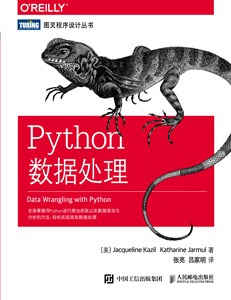2018-08-23 18:41:45 a40850273 阅读数 333
• ###### Python数据殿堂：数据分析与数据可视化

【入门基础+轻实战演示】【讲授方式轻松幽默、有趣不枯燥、案例与实操结合，与相关课程差异化】利用python进行数据处理、 分析，并结合大量具体的例子，对每个知识进行实战讲解，本课程通过大量练习和案例对各个知识点技能进行详细讲解。通过本 课程，学生将懂得pandas、numpy、matplotlib等数据分析工具；通过实战，学生将了解标准的数据分析流程，学会使用可视化的 方法展示数据及结果。

6584 人正在学习 去看看 刘英

# Python 数据处理

### 数据的读取与保存

``````import pandas as pd

### DataFrame 按列差分

``d_vel = abs(data.diff()).fillna(method='bfill')``

### 数据插值

interp1d 函数通过传入的横纵坐标数据拟合并返回一个函数。这个函数可以接受一个序列作为输入，并返回相应大小的拟合插值。

``````from scipy.interpolate import interp1d

function = interp1d(range(N), data[i], kind='quadratic') # interp1d(x, y) 一维插值
fdata = function(np.linspace(0, N-1, INSERT_N * N))``````

``````from scipy import interp, arange, exp

x = arange(0, 10)
y = exp(-x/3.0)

interp([9, 10], x, y)
# array([0.04978707, 0.04978707])
``````

### 中值滤波

``````import scipy.signal as signal

x_med = signal.medfilt(x, 3) # 核为3``````

### 分位数计算

percentile 函数根据传入的数据，给出对应 25%、50%和75%的分位数

``````import numpy as np

per = np.percentile(x, [25, 50, 75])``````

### 对数组前 k 个极值划分

argpartition 函数根据传入的数据，对数组中的元素值进行划分，并返回划分之后对应元素的下标

``````import numpy as np

index = np.argpartition(arr, -3)  # 返回数组中后 3 位存放最大的 3 个元素
idx = index[-3:]  # 取得最大 3 个数的下标
``````

### 离散信号的极值点

``````import scipy.signal as signal

extreme_geater = signal.argrelextrema(data, np.greater) # 给出的是位置标号
extreme_less = signal.argrelextrema(data, np.less)``````

### 绘图使用两个独立的坐标轴

``````import matplotlib.pyplot as plt

plt.figure()
ax1 = plt.subplot(111)
ax1.plot(range(N), f_data)
ax2 = ax1.twinx() # 独立两个y轴，x轴用twiny()
ax2.plot(range(N), data)``````

python 超大数据处理 相关内容

2019-08-17 01:28:50 qq_41821116 阅读数 100
• ###### Python数据殿堂：数据分析与数据可视化

【入门基础+轻实战演示】【讲授方式轻松幽默、有趣不枯燥、案例与实操结合，与相关课程差异化】利用python进行数据处理、 分析，并结合大量具体的例子，对每个知识进行实战讲解，本课程通过大量练习和案例对各个知识点技能进行详细讲解。通过本 课程，学生将懂得pandas、numpy、matplotlib等数据分析工具；通过实战，学生将了解标准的数据分析流程，学会使用可视化的 方法展示数据及结果。

6584 人正在学习 去看看 刘英

## 第一步：基础IO操作

### 文本对象读写

``````fp = open('file_name', 'mode')  #其实就是生成一个文件对象
...
...
fp.close()
``````

mode可选，会有不同的效果：

``````    'r'       open for reading (default)
'w'       open for writing, truncating the file first
'x'       create a new file and open it for writing
'a'       open for writing, appending to the end of the file if it exists
'b'       binary mode
't'       text mode (default)
'+'       open a disk file for updating (reading and writing)

``````

``````>>> with open('workfile', 'r') as f:
>>> f.closed
True
``````

``````f.read() #一次性读取整个文件

f.write(astr)
``````

``````>>> for line in f.readlines():
...     print(line, end='')
...

``````

``````>>> for line in f:
...     print(line, end='')
...

``````

python对象的序列化：

``````#对象序列化到本地
with open('a_list.txt', 'w') as f:
a_list = [1,2,3,4]
json.dump(a_list, f)
#这样就将a_list这个对象存储到了本地的一个文本文件中
#下次直接从这个文本文件中加载，就能获得a_list这个对象
#json.dump(ensure_ascii=True)默认为ensure_ascii=True，这样会让存储到本地文件中的中文显示‘乱码’。改成True就好了。
``````
``````#从本地加载一个对象
with open('a_list.txt') as f:
``````

json还有`.loads()``dumps()`的方法，可以将信息从字符串python对象之间来回转换。

`pickle`的使用方法和`json`类似，不再赘述。

### 图像读写

``````#打开图像，获取图像的一些数据：
from PIL import Image
#获取图像对象
img = Image.open('test.png')
#获取图片高度、宽度
h, w = img.size
#用默认图片查看软件查看图片：
img.show()
#查看图片文件名：
img.filename
#获取图片的像素：
img.getpixel((heighth, weighth))
#如果图片是RGB三维的，那么就会得到如下格式的数据：(62, 131, 200)
#如果图片是灰度图，会得到一个数值
#如果是黑白图，会得到0或者1

#将RGB图片转换为二值图像
img_gray = img.convert('L') #先转化为灰白图
tab = [0 if i < 160 else 1 for i in range(256)]
black_white_img = img_gray.point(tab) #灰白图转化为黑白图

#图片的保存：
img.save('file_name.png')
``````

### 读写csv文件

``````csv.reader(iterable, delimiter=',')
csv.writer(fileobj, delimiter=',')
#打开了读、写器之后，就可以方便的读写csv文件了。
``````

csv模块只适合简单读取、遍历.csv文件，不适合做复杂的筛选、修改工作。后者这样的工作要交给pandas这个神器。所以这里暂时先一笔带过。

## 第二步：掌握更高效的数据处理方法

### numpy这个利器你值得拥有

#### 基本数据格式和操作

• 基本整形：np.int8、np.int16、np.int32、np.int64等
• 基本浮点型：np.float16、np.float32、np.float64等
• 加减乘除：np.add()、np.subtract()、np.multiply()、np.divide()。或者直接用`+ - * /`这些符号也可以。

#### numpy数组

numpy的核心是`ndarray`对象，它是封装了python基本数据类型的n维数组。numpy数组有如下几个特点：

• 无法修改一个已经创建好的numpy数组
• 一个numpy数组所有数据类型相同
• numpy数组包括大量的矩阵运算方法
##### numpy数组方便在何处？

1. python数组实现：
``````a = [1, 2, 3]
b = [4, 5, 6]
ans = [i*j for i, j in zip(a, b)]
``````
1. numpy数组实现：
``````a = np.array([1,2,3])
b = np.array([4,5,6])
ans = a*b
``````

np数组让计算更接近数学符号！np的计算面向np的数组，而不是其中的一个一个元素。

np数组的属性：

• ndarrary.dtype：元素类型
• nparray.shape：数组的结构
• nparray.size：元素个数
• nparray.real：元素实部
• nparray.imag：元素虚部
• nparray.astype()：修改元素类型
• nparray.reshape()：重新修改元素的结构，但是修改后元素的个数不能改变
##### 创建np数组：
1. 创建简单数组

``````np.array([1,2,3,4]).reshape((2,2)).astype(np.float)
np.zeros(shape, dtype=float) #纯零矩阵
np.ones(shape, dtype=float) #纯幺矩阵
np.eye(N, dtype=float) #单位矩阵
np.empty(shape, dtype=float) #空阵
``````
2. 创建随机矩阵

• np.random.rand(axis1, axis2, axis3, …, axisn)
uniform distribution over [0, 1)
• np.random.ranint(low, high=None, size=None, dtype=l)
uniform distribution over [low, high) of size
• np.random.randn(axis1, axis2, axis3, …, axisn)
standard normal distribution. u=0, sigma=1
• np.random.random(size=None)
uniform distribution over [0, 1)
• np.random.random_integers(low, high=None, size=None)
discrete uniform distribution over [low, hgih] of type np.int
1. 在指定范围创建数组

``````np.arange(low, high, step=) #开区间，包括low，不包括high
np.linsapce(low, high, num=) #闭区间，包括low, high
``````
2. 从已有数组创建新数组

``````np.asarray(array, dtype=None) #获取一个数组array的副本，拥有和array相同的地址
np.empty_like(a) #创建和a相同形状的一个空数组
np.zeros_like(a) #创建和a相同形状的一个全零数组
np.ones_like(a) #创建和a相同形状的一个全幺数组
``````
3. 构造复杂数组

整个数组的重复

``````np.tile(a, n)
#如果a=array([1, 2, 3])， 用此方法重复3次后，得到array([1, 2, 3, 1, 2, 3, 1, 2, 3])
``````

数组内各个元素的重复

``````np.repeat(a, 3)
或者
a.repeat(3)
#如果a=array([1, 2, 3])， 用此方法重复3次后，得到array([1, 1, 1, 2, 2, 2, 3, 3, 3])
``````

数组的合并
append

``````np.append([1,2,3],[[4,5,6],[7,8,9]])
>>>array([1, 2, 3, 4, 5, 6, 7, 8, 9])
np.append([[1,2,3]], [[4,5,6]], axis=0)
>>>array([[1, 2, 3],
[4, 5, 6]])
np.append([[1,2,3]], [[4,5,6]], axis=1)
>>>array([[1, 2, 3, 4, 5, 6]])
``````

concatenate与append基本相似，只不过是把两个合并对象合并到了一个元组内

``````np.concatenate(([[1,2,3]], [[4,5,6]]), axis=0)
>>>array([[1, 2, 3],
[4, 5, 6]])
``````

数组拆分

### pandas让你提取数据从此不犯难

panel：面板数据——计量经济学

#### 基础处理

##### pandas核心数据结构

• DataFrame
DataFrame相当于有行索引和列索引的二维表。
常用属性:
• Panel
• Series

## 第四步：深度学习之旅

python 超大数据处理 相关内容

2017-06-28 16:31:05 qq_28172757 阅读数 1090
• ###### Python数据殿堂：数据分析与数据可视化

【入门基础+轻实战演示】【讲授方式轻松幽默、有趣不枯燥、案例与实操结合，与相关课程差异化】利用python进行数据处理、 分析，并结合大量具体的例子，对每个知识进行实战讲解，本课程通过大量练习和案例对各个知识点技能进行详细讲解。通过本 课程，学生将懂得pandas、numpy、matplotlib等数据分析工具；通过实战，学生将了解标准的数据分析流程，学会使用可视化的 方法展示数据及结果。

6584 人正在学习 去看看 刘英

判断给出的字符串中的括号是否合理，若合理，将括号里面的括号删除，其他内容不变，并返回

```# -*- coding: utf8 -*-
class manager(object):
def __init__(self):
self.symbols_location = list()
self.symbols_count = list()

def islegal(self, s):
'''
判断给出的字符串中括号是否合法
:param s: 字符串
:return: True or False
'''
SYMBOLS = {'}': '{', ']': '[', ')': '('}
SYMBOLS_L, SYMBOLS_R = SYMBOLS.values(), SYMBOLS.keys()
arr = []
for c in s:
if c in SYMBOLS_L:
# 左符号入栈
arr.append(c)
elif c in SYMBOLS_R:
# 右符号要么出栈，要么匹配失败
if arr and arr[-1] == SYMBOLS[c]:
arr.pop()
else:
return False
return not arr

def check_symbol_location(self, str):
'''
检查出所有左括号和右括号的位置
左括号计数加1，右括号计算减一，将每次的计数都记录下来，[1, 0]表示刚好出现了成对括号()
:param str: 给出的字符串
:return:
'''
count = 0
for i in range(len(str)):
if str[i] == '(':
self.symbols_location.append(i)
count += 1
self.symbols_count.append(count)
elif str[i] == ')':
self.symbols_location.append(i)
count -= 1
self.symbols_count.append(count)

def symbol_clear_index(self):
'''
遍历括号计算列表，若括号为包含关系时，列表中连续2个值一定是左边的值大于右边的值，且右边的值不等于0
:return: 找出括号包含关系时，子括号在字符串中的索引位置
'''
for i in range(len(self.symbols_count)-1):
if self.symbols_count[i] > self.symbols_count[i+1] and self.symbols_count[i+1]:
index = [self.symbols_location[i], self.symbols_location[i+1]]
del self.symbols_location[i]
del self.symbols_location[i]
del self.symbols_count[i]
del self.symbols_count[i]
return index

def data_manager(self, str):
if self.islegal(str):
s = ''
all_del_symbol = list()
self.check_symbol_location(str)
symbol_index = self.symbol_clear_index()
while symbol_index:
# 循环判断返回的值，若为真，再次调用symbol_clear_index()，直到返回的索引为空
all_del_symbol.extend(symbol_index)
# 将每次返回的需要删除的括号在字符串中的索引位置记录下来
symbol_index = self.symbol_clear_index()
for k, v in enumerate(str):
# 遍历字符串的位置k和值v，将不在需要删除列表中的值记录下来
if k not in all_del_symbol:
s += v
return '整理后结果为： %s'%s
else:
return '该字符串的括号不合法'

if __name__ == "__main__":
manager = manager()
print(manager.data_manager("1 - 2*(17-(60-30+(-40*2/5)*9-2*5/3+7/3*99/4*2998+10*568/14))-(-4*3)/(16-3*2)"))
print(manager.data_manager("1 - 2*(17-(60-30+(-40*(2)/5)*9-2*5/3+7/3*99/4*2998+10*568/14))-(-4*3)/(16-3*2)"))
print(manager.data_manager("1 - 2*(17-60）-30+(-40*2/"))```

整理后结果为： 1 - 2*(17-60-30+-40*2/5*9-2*5/3+7/3*99/4*2998+10*568/14)-(-4*3)/(16-3*2)
整理后结果为： 1 - 2*(17-60-30+-40*2/5*9-2*5/3+7/3*99/4*2998+10*568/14)-(-4*3)/(16-3*2)
该字符串的括号不合法

python 超大数据处理 相关内容

2018-01-04 21:15:15 shaxiaozilove 阅读数 1468
• ###### Python数据殿堂：数据分析与数据可视化

【入门基础+轻实战演示】【讲授方式轻松幽默、有趣不枯燥、案例与实操结合，与相关课程差异化】利用python进行数据处理、 分析，并结合大量具体的例子，对每个知识进行实战讲解，本课程通过大量练习和案例对各个知识点技能进行详细讲解。通过本 课程，学生将懂得pandas、numpy、matplotlib等数据分析工具；通过实战，学生将了解标准的数据分析流程，学会使用可视化的 方法展示数据及结果。

6584 人正在学习 去看看 刘英

1、安装python（https://www.python.org/

2、安装python模块管理工具pip
python 3.x安装后，默认已经包含pip（在/Scripts路径下），要想pip对python的包进行管理，需要将Scripts路径添加到系统环境变量Path下面。配置完成后，在cmd中输入pip可查看相关的指令说明。

3、安装地理数据处理的常用模块，主要模块如下：
GDAL/OGR：用于阅读和编写地理空间数据，具体安装方法详见4中的说明；
Numpy：基本的Python数组处理模块，可通过 pip install numpy快速安装；
Matplotlib：用于绘制图像数据，安装方式同 numpy；
Scipy：一个科学计算模块，安装方式同上；
Pyproj：一个用于PROJ.4制图投影库的Python包容器，安装方法后续说明
Folium：用于使用Python制作Leaflet.js地图，通过pip install folium安装；
Spectral Python：用于处理高光谱图像数据，安装方法后续说明
scikit-learn：用于数据分析，安装方法后续说明
Mapnik，用于制作美丽的地图，安装方法后续说明

4、GDAL的安装
(1)快速安装方式

pip install GDAL-2.1.3-cp35-cp35m-win_amd64.whl安装即可

from osgeo import gdal
gdal.__version__

（2）常规安装

python 超大数据处理 相关内容

2019-05-27 19:13:25 Charein 阅读数 1396
• ###### Python数据殿堂：数据分析与数据可视化

【入门基础+轻实战演示】【讲授方式轻松幽默、有趣不枯燥、案例与实操结合，与相关课程差异化】利用python进行数据处理、 分析，并结合大量具体的例子，对每个知识进行实战讲解，本课程通过大量练习和案例对各个知识点技能进行详细讲解。通过本 课程，学生将懂得pandas、numpy、matplotlib等数据分析工具；通过实战，学生将了解标准的数据分析流程，学会使用可视化的 方法展示数据及结果。

6584 人正在学习 去看看 刘英### 本书特色

• 快速了解Python基本语法、数据类型和语言概念
• 概述数据的获取与存储方式
• 清洗数据并格式化，以消除数据集中的重复值与错误
• 学习何时对数据进行标准化，何时对数据清理进行测试并将其脚本化
• 使用Scrapy写网络爬虫
• 利用新的Python库和技术对数据集进行探索与分析
• 使用Python解决方案将整个数据处理过程自动化

“如果你一直感觉电子表格（甚至关系型数据库）无法回答你想要提出的问题，或者除这些工具之外你准备进一步学习，那么这本书非常适合你。我一直在等待这本书的出现。”

——Derek Willis，ProPublica新闻应用开发者，OpenElections联合创始人

“所有新手数据科学家、数据工程师或其他技术方面的数据专家都应该读一读这本实践指南。数据处理领域正需要这样一本书，真希望我第一次开始用Python处理数据时就能有它指导。”

——Tyrone Grandison博士，Proficiency Labs Intl. CEO

### 目录

O’Reilly Media, Inc.介绍

### 下载

https://pan.baidu.com/s/1B2H_CxsXfnjRouxU6bCTRw

python 超大数据处理 相关内容